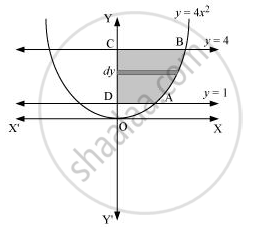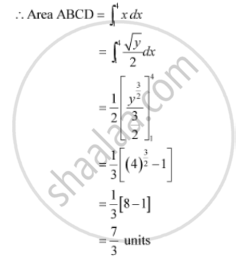Share

# Find the Area of the Region Lying in the First Quadrant and Bounded by Y = 4x2, X = 0, Y = 1 and Y = 4 - CBSE (Commerce) Class 12 - Mathematics

#### Question

Find the area of the region lying in the first quadrant and bounded by y = 4x2x = 0, y = 1 and = 4

#### Solution

The area in the first quadrant bounded by y = 4x2x = 0, y = 1, and = 4 is represented by the shaded area ABCDA asIs there an error in this question or solution?

#### APPEARS IN

NCERT Solution for Mathematics Textbook for Class 12 (2018 to Current)
Chapter 8: Application of Integrals
Q: 3 | Page no. 375
Solution Find the Area of the Region Lying in the First Quadrant and Bounded by Y = 4x2, X = 0, Y = 1 and Y = 4 Concept: Area Under Simple Curves.
S